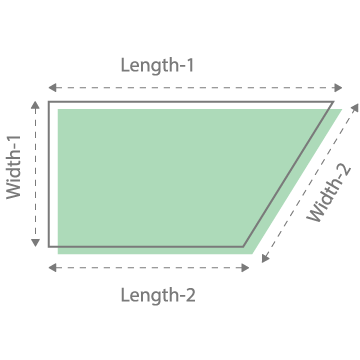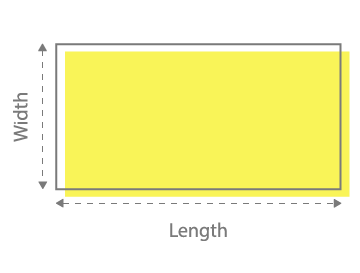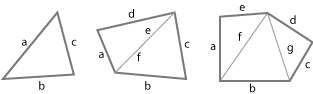X

## Finding area of irregular shapes / area.

Remember, the input can only be in feet (ft), inches (in), yards (yd), centimetres (cm), millimetres (mm) and metres (m) but never a combination of two different units!. . If you have inputs in different units of measurement. Please refer to our conversion table below.

Formula

Area = b × h# Understanding the area of irregular shape:

### What is the difference between regular and irregular shape?

Regular Shape: The sides and angles of regular shapes are all equal.

Irregular Shape: The sides and angles of an irregular shape can be of any length and size.Regular Shape

Irregular Shape

### How to calculate area of an irregular shape?

Now that we know the difference between regular and irregular shapes. Let’s make our calculation process simple by dividing the irregular shape into regular shapes. Check example below, here we have divided the shapes into triangles which is a regular shape.Then, you can easily calculate the area of each triangle by using the Heron’s Formula.After calculating area of each triangle, simply add all of them to find the area of an irregular shape.

### How to use irregular area calculator?

Step 1: Measure all sides of the area in one unit (Feet, Meter, Inches or any other).

Step 2: Enter length of horizontal sides into Length 1 and Length 2. And Width of the vertical sides into Width 1 and Width 2. (See image above).

Step 3: Press calculate button. The output our calculator provides is independent of the unit of measurement. For example, If you have entered values in feet. The output will always be in feet.

Our Formula: Area = b × h

Abbreviations of unit area:

Ft2 = Square feet

In2 = Square Inches

Yd2 = Square Yards

Cm2 = Square Centimeters

Mm2 = Square Millimeters

M2 = Square Meters

# How to find the area of a parallelogram?

Step 1: Determine all the sides of irregular shape, Make sure all the sides are in same unit.

Step 2: Draw the area on a piece of paper using the measurements you obtained. Remember your drawing is to scale.

Step 3: Divide the drawing into different shapes. The easy ones are Square and rectangle, circles and triangle could be a bit tricky.

Step 4: Calculate the area of each shape using our Square footage calculator. For rectangles and squares, multiply width by height. For triangles, multiply the base by the height and then divide by 2. For circles, multiply the radius square by 3.14 (pi). The radius of a circle is the distance from its center point to any other point on the edge of the circle.

Step 5: Add the areas of all the individual shapes to find the total square footage.

Our Formula: Area = b × h

Conversion factors:

To convert among square feet, square inches, square yards, square centimetres, square millimetres and square meters you can utilize the following conversion table.

 Square feet to yards multiply ft2 by 0.11111 to get yd2 Square feet to meters multiply ft2 by 0.092903 to get m2 Square yards to square feet multiply yd2 by 9 to get ft2 Square yards to square meters multiply yd2 by 0.836127 to get m2 Square meters to square feet multiply m2 by 10.7639 to get ft2 Square meters to square yards multiply m2 by 1.19599 to get yd2 Square meters to square millimetres multiply the m2 value by 1000000 to get mm2 Square meters to square centimetres multiply the m2 value by 10 000 to get cm2 Square centimetres to square metres multiply the cm2 value by 0.0001 to get mm2 Square centimetres to square millimetres multiply the cm2 value by 100 to get mm2 Square millimetres to square centimetres multiply the mm2 value by 0.000001 to get cm2 Square millimetres to square metres multiply the mm2 value by 1000000 to get m2